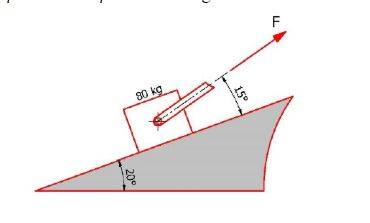# What is the minimum force required to move the box?

thomovich
Homework Statement:
What is the minim Force F necessary to make the crate start moving up the incline?
Relevant Equations:
F_push=mgsin(ø)+F_f
F_f= µ_s mgcos(ø)
Problem Statement: What is the minim Force F necessary to make the crate start moving up the incline?
Relevant Equations: F_push=mgsin(ø)+F_f
F_f= µ_s mgcos(ø)

My values
m = 80kg
ø = 20
Fø = 15
static friction = 0,5
constant friction = 0,4
F _push = 80kg * 9.8 m/s^2 *sin(20) + 0,5 * 80kg * 9.8 m/s^2 * cos(20)
This gives 636,503N
Because the force is coming at an angle i divide my Newtons with cos
636,503N/cos(15) = 658,956 N required to make the crate move.
Attached below is the photo of the drawing.Mentor
F_f= µ_s mgcos(ø)
Careful! A more accurate version would be: F_f= µ_s N, where N is the normal force. (Since the applied force is at an angle, it will affect the normal force.)

thomovich
Okay, that is true but in order to find my N i need to F and my task is to find F, can you lead in the direction of what equations I am looking for to solve this problem? Cause at this moment i don't know where to go forward that's why i went to the forum.

Homework Helper
Gold Member
2022 Award
Okay, that is true but in order to find my N i need to F and my task is to find F, can you lead in the direction of what equations I am looking for to solve this problem? Cause at this moment i don't know where to go forward that's why i went to the forum.
This is where algebra is useful. I can see you have been taught to plug in the numbers but that makes it difficult in this case.

You should keep ##F## and ##N## as variables and look at all the forces on the block.

•CWatters